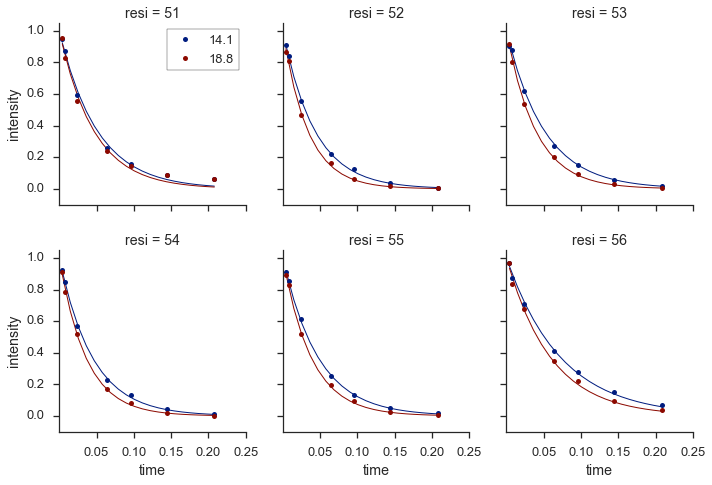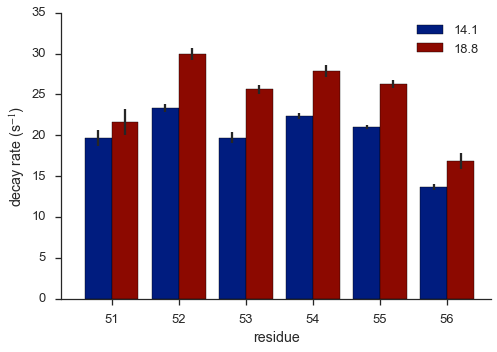# pdLSR: Pandas-aware least squares regression

I have a new Python project I would like to share with the community. Actually, this project isn't so new. I developed an initial version about two years before completing my postdoctoral research, and it has undergone various revisions over the past three years. Having finally made time to give it the clean-up it needed,1 I am excited to share it on GitHub.

## Overview

pdLSR is a library for performing least squares minimization. It attempts to seamlessly incorporate this task in a Pandas-focused workflow. Input data are expected in dataframes, and multiple regressions can be performed using functionality similar to Pandas groupby. Results are returned as grouped dataframes and include best-fit parameters, statistics, residuals, and more. The results can be easily visualized using seaborn.

pdLSR currently utilizes lmfit, a flexible and powerful library for least squares minimization, which in turn, makes use of scipy.optimize.leastsq. I began using lmfit because it is one of the few libraries that supports non-linear least squares regression, which is commonly used in the natural sciences. I also like the flexibility it offers for testing different modeling scenarios and the variety of assessment statistics it provides. However, I found myself writing many for loops to perform regressions on groups of data and aggregate the resulting output. Simplification of this task was my inspiration for writing pdLSR.

pdLSR is related to libraries such as statsmodels and scikit-learn that provide linear regression functions that operate on dataframes. However, these libraries don't directly support grouping operations on dataframes.

The aggregation of minimization output parameters that is performed by pdLSR has many similarities to the R library broom, which is written by David Robinson and with whom I had an excellent conversation about our two libraries. broom is more general in its ability to accept input from many minimizers, and I think expanding pdLSR in this fashion, for compatibility with statsmodels and scikit-learn for example, could be useful in the future.

## Minimization setup¶

To demonstrate how to use pdLSR, I will be using some sample scientific data. This data is nuclear magnetic resonance (NMR) data acquired at two different magnetic field strengths (14.1 and 18.8 T) on the DNA-binding region of a transcription factor called GCN4.

There are six amino acids in the enclosed data set (numbered 51 - 56), so using amino acid residue (resi) and magnetic field (field) as the groupby columns means we will need to perform twelve regressions.

In :
data = pd.read_csv('GCN4_twofield.tsv', sep='\t')

resi	field	time	intensity
51	14.1	0.004	1624.219428
51	14.1	0.008	1491.46728
51	14.1	0.024	1022.717456
51	14.1	0.064	448.038543
51	14.1	0.096	270.116745
51	14.1	0.144	148.671651
51	14.1	0.208	103.322608
52	14.1	0.004	1614.38584
52	14.1	0.008	1500.47205


We will be analyzing this data to determine the rate of exponential decay as a function of time for every amino acid residue at each of the two magnetic field strengths. The equation looks like this:

$I_{(t)} = I_{(0)} \space e^{(-R * t)}$

where $I_{(0)}$ is the initial intensity, $I_{(t)}$ is the intensity at time $t$, and $R$ is the exponential decay rate. We have $I_{(t)}$ and $t$ from the data above and will use NLS to determine $I_{(0)}$ and $R$.

## Regression and prediction¶

Parameters for pdLSR are entered as a list of dictionaries with the format being similar to that used by lmfit.

Performing the regression is quite simple--just call the class pdLSR with input parameters set. The fit method performs the regression and predict calculates a best-fit line at higher resolution for plotting.

In :
params = [{'name':'inten',
'value':np.asarray(data.groupby(['resi', 'field']).intensity.max()),
'vary':True},
{'name':'rate',
'value':20.0,
'vary':True}]

minimizer_kwargs = {'params':params,
'method':'leastsq',
'sigma':0.95,

fit_data = pdLSR.pdLSR(data, exponential_decay,
groupby=['resi', 'field'],
xname='time', yname='intensity',
minimizer='lmfit',
minimizer_kwargs=minimizer_kwargs)
fit_data.fit()
fit_data.predict()


## Results¶

From these simple commands, five output tables are created:

• data for the input data, calculated data, and residuals
• results that contains the best-fit parameters and estimation of their error
• stats for statistics related to the regression, such as chi-squared and AIC
• model that contains a best-fit line created by the predict method
• covar that contains the covariance matrices

Let's take a look at a couple of these tables. The results table contains best-fit parameters, their standard errors, and confidence intervals.

In :
fit_data.results.head(n=4)

Out:
inten rate
value stderr ci0.95_lo ci0.95_hi value stderr ci0.95_lo ci0.95_hi
resi field
51 14.10 1714.80 37.87 1656.39 1833.26 19.65 1.02 17.91 22.46
18.80 24122.68 689.03 22988.09 25953.74 21.64 1.58 18.83 25.55
52 14.10 1778.90 17.87 1746.80 1814.00 23.35 0.49 22.39 24.36
18.80 23889.26 232.87 23351.24 24329.26 29.96 0.79 28.27 31.59

The stats table contains the following statistics for each of the regression groups:

• Number of observations (nobs)
• Number of fit parameters (npar)
• Degrees of freedom (dof)
• Chi-squared (chisqr)
• Reduced chi-squared (redchi)
• Akaike information criterion (aic)
• Bayesian information criterion (bic)
In :
fit_data.stats.head(n=4)

Out:
nobs npar dof chisqr r_chisqr aic bic
resi field
51 14.10 7 2 5 46189329.09 9237865.82 116.27 116.16
18.80 7 2 5 5181283778043.33 1036256755608.67 197.67 197.56
52 14.10 7 2 5 2013267.84 402653.57 94.34 94.23
18.80 7 2 5 184908562051.39 36981712410.28 174.34 174.23

It is also easy to access the covariance matrix for calculations.

In :
fit_data.covar.loc[(51, 14.1)]

Out:
row col covar
resi field
51 14.10 0 0 1434.41
14.10 0 1 25.19
14.10 1 0 25.19
14.10 1 1 1.04
In :
fit_data.pivot_covar().loc[(51, 14.1)].values

Out:
array([[  1.43e+03,   2.52e+01],
[  2.52e+01,   1.04e+00]])

## Visualization

The results can be visualized in facet plots with Seaborn. To make it easier to view the data, all intensities have been normalized.

In :
plot_data = pd.concat([fit_data.data,
fit_data.model], axis=0).reset_index()

colors = sns.color_palette()

palette = {14.1:colors, 18.8:colors}

grid = sns.FacetGrid(plot_data, col='resi', hue='field', palette=palette,
col_wrap=3, size=2.0, aspect=0.75,
sharey=True, despine=True)

grid.map(plt.plot, 'xcalc', 'ycalc', marker='', ls='-', lw=1.0)
grid.map(plt.plot, 'time', 'intensity', marker='o', ms=5, ls='')

grid.set(xticks=np.linspace(0.05, 0.25, 5),
ylim=(-0.1, 1.05))

ax = grid.axes
legend = ax.get_legend_handles_labels()
ax.legend(legend[2:], legend[2:], loc=0, frameon=True)

f = plt.gcf()
f.set_size_inches(12,8)Just for fun, here's a bar graph of the decay rates determined from NLS.

In :
plot_data = (fit_data.results
.sort_index(axis=1)
.loc[:,('rate',['value','stderr'])]
)
plot_data.columns = plot_data.columns.droplevel(0)
plot_data.reset_index(inplace=True)

fig = plt.figure()
fig.set_size_inches(7,5)
ax = plt.axes()

palette = [colors, colors]

for pos, (field, dat) in enumerate(plot_data.groupby('field')):
_ = dat.plot('resi', 'value', yerr='stderr',
kind='bar', label=field, color=palette[pos],
position=(-pos)+1, ax=ax, width=0.4)

ax.set_ylabel('decay rate (s$^{-1}$)')
ax.set_xlabel('residue')
ax.set_xlim(ax.get_xlim()-0.5, ax.get_xlim())
plt.xticks(rotation=0)

sns.despine()
plt.tight_layout()## Conclusion

Easy right? Using pdLSR over the past few years has made my Python-based analytical workflows much smoother. Let me know how it works for you if you decide to try it!

If you are interested in trying pdLSR, you can do so without even installing it. There is a live demo available on GitHub. Click here and navigate to pdLSR --> demo --> pdLSR_demo.ipynb.

The package can be installed in three different ways:

This post was written in a Jupyter notebook, which can be downloaded and viewed statically here.

1. And with a helpful nudge in the form of the excellent data science bootcamp I'm currently attending. Stay tuned for more about that!